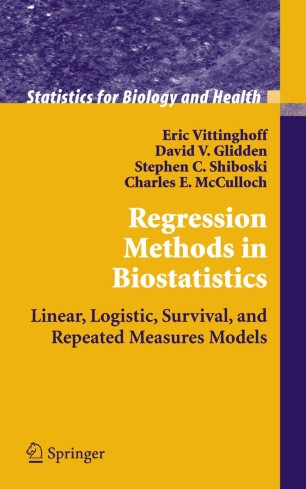# Regression Methods in Biostatistics

## Linear, Logistic, Survival, and Repeated Measures Models

• Eric Vittinghoff
• Stephen C. Shiboski
• David V. Glidden
• Charles E. McCullochBook

Part of the Statistics for Biology and Health book series (SBH)

1. Front Matter
Pages I-XV
2. Pages 1-5
3. Pages 7-27
4. Pages 29-67
5. Pages 69-131
6. Pages 133-156
7. Pages 157-209
8. Pages 211-252
9. Pages 253-289
10. Pages 291-303
11. Pages 305-316
12. Pages 317-322
13. Back Matter
Pages 323-340

### Introduction

This new book provides a unified, in-depth, readable introduction to the multipredictor regression methods most widely used in biostatistics: linear models for continuous outcomes, logistic models for binary outcomes, the Cox model for right-censored survival times, repeated-measures models for longitudinal and hierarchical outcomes, and generalized linear models for counts and other outcomes.

Treating these topics together takes advantage of all they have in common. The authors point out the many-shared elements in the methods they present for selecting, estimating, checking, and interpreting each of these models. They also show that these regression methods deal with confounding, mediation, and interaction of causal effects in essentially the same way.

The examples, analyzed using Stata, are drawn from the biomedical context but generalize to other areas of application. While a first course in statistics is assumed, a chapter reviewing basic statistical methods is included. Some advanced topics are covered but the presentation remains intuitive. A brief introduction to regression analysis of complex surveys and notes for further reading are provided. For many students and researchers learning to use these methods, this one book may be all they need to conduct and interpret multipredictor regression analyses.

The authors are on the faculty in the Division of Biostatistics, Department of Epidemiology and Biostatistics, University of California, San Francisco, and are authors or co-authors of more than 200 methodological as well as applied papers in the biological and biomedical sciences. The senior author, Charles E. McCulloch, is head of the Division and author of Generalized Linear Mixed Models (2003), Generalized, Linear, and Mixed Models (2000), and Variance Components (1992).

### Keywords

Generalized linear model Logistic Regression Regression analysis Stata Survival analysis Variance applied regression methods for biomedical research confounding, mediation, and interaction data analysis linear regression linear, logistic, generalized linear, survival (Cox), GEE, a model selection and checking statistical computing with STATA statistics

#### Authors and affiliations

• Eric Vittinghoff
• 1
• Stephen C. Shiboski
• 1
• David V. Glidden
• 1
• Charles E. McCulloch
• 1
1. 1.Department of Epidemiology and BiostatisticsUniversity of CaliforniaSan FranciscoUSA

### Bibliographic information

• DOI https://doi.org/10.1007/b138825